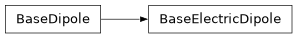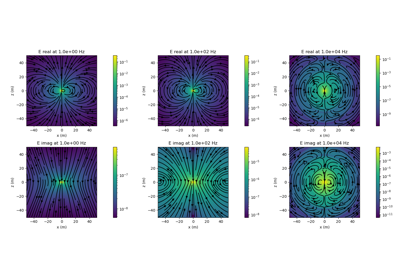# geoana.em.BaseElectricDipole#class geoana.em.BaseElectricDipole(length=1.0, current=1.0, **kwargs)#

Base class for electric current dipoles.

The BaseElectricDipole class defines the basic properties for electric current dipoles. Where $$I$$ is the current and $$d\mathbf{s}$$ defines the direction and length of the dipole, the current dipole is defined as:

$\mathbf{j_s}(\mathbf{r}) = Id \mathbf{s} \, \delta(|\mathbf{r} - \mathbf{r_s}|)$

where $$\mathbf{r_s}$$ is the location of the source. For more, visit EM GeoSci .

Parameters
lengthfloat, int

Length of the electric current dipole (m). Default is 1 m.

currentfloat, int

Current of the electric current dipole (A). Default is 1 A.

Attributes

 current Current in the electric current dipole (A) length Length of the electric current dipole (m) location Location of the dipole orientation Orientation of the dipole as a normalized vector

Methods

 Cross products between a gridded set of vectors and the orientation of the source. distance(xyz) Scalar distance from dipole to a set of gridded xyz locations Dot product between the orientation of the source and a gridded set of vectors. Vector distance to a set of gridded xyz locations.

## Galleries and Tutorials using geoana.em.BaseElectricDipole#Electric Dipole in a Whole Space: Frequency Domain

Electric Dipole in a Whole Space: Frequency Domain